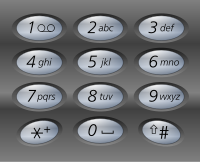# GeetCode Hub

Given a string containing digits from `2-9` inclusive, return all possible letter combinations that the number could represent. Return the answer in any order.

A mapping of digit to letters (just like on the telephone buttons) is given below. Note that 1 does not map to any letters.Example 1:

```Input: digits = "23"
```

Example 2:

```Input: digits = ""
Output: []
```

Example 3:

```Input: digits = "2"
Output: ["a","b","c"]
```

Constraints:

• `0 <= digits.length <= 4`
• `digits[i]` is a digit in the range `['2', '9']`.

class Solution { public List<String> letterCombinations(String digits) { List<String> res = new ArrayList<String>(); if(digits == null){ return res; } int len = digits.length(); if(len == 0){ return res; } String[] map = new String[]{ "", "", "abc", "def", "ghi", "jkl", "mno", "pqrs", "tuv", "wxyz" }; letterCombinations(digits, 0, "", map, res); return res; } private void letterCombinations( String digits, int start, String asf, String[] map, List<String> res){ if(start == digits.length()){ res.add(asf); return; } int val = digits.charAt(start)-'0'; //'0' - 48 , '9' 57 - 48 => 9 String options = map[val]; for(int i=0;i<options.length();i++){ letterCombinations(digits, start+1, asf+options.charAt(i), map, res); } } }

The main thing to know for this question is the Level and Option approach in Recursion. Once you know the concept then it is very easy to code this.

Time Complexity: O(4^n)  // we are having a maximum of 4 options possible at each level and total n levels

Space Complexity: O(n)  // for system recursion stack